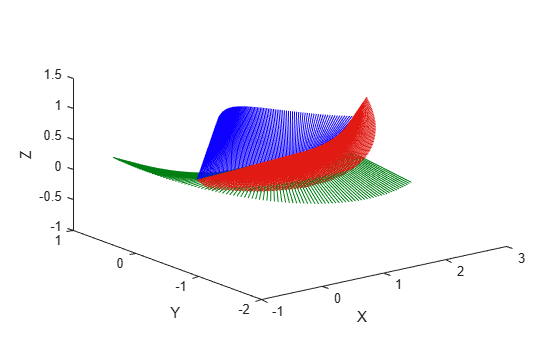Documentation

transformtraj

Generate trajectories between two transformations

Description

example

[tforms,vel,acc] = transformtraj(T0,TF,tInterval,tSamples) generates a trajectory that interpolates between two 4-by-4 homogeneous transformations, T0 and TF, with points based on the time interval and given time samples.

[tforms,vel,acc] = transformtraj(T0,TF,tInterval,tSamples,Name,Value) specifies additional parameters using Name,Value pair arguments.

Examples

collapse all

Build transformations from two orientations and positions. Specifiy the time interval and vector of times for interpolating.

t0 = axang2tform([0 1 1 pi/4])*trvec2tform([0 0 0]);
tF = axang2tform([1 0 1 6*pi/5])*trvec2tform([1 1 1]);
tInterval = [0 1];
tvec = 0:0.01:1;

Interpolate between the points. Plot the trajectory using plotTransforms. Convert the transformations to quaternion rotations and linear transitions. The figure shows all the intermediate transformations of the coordinate frame.

[tfInterp, v1, a1] = transformtraj(t0,tF,tInterval,tvec);

rotations = tform2quat(tfInterp);
translations = tform2trvec(tfInterp);

plotTransforms(translations,rotations)
xlabel('X')
ylabel('Y')
zlabel('Z')Input Arguments

collapse all

Initial transformation, specified as a 4-by-4 homogeneous transformation. The function generates a trajectory that starts at the initial transformation, T0, and goes to the final transformation, TF.

Data Types: single | double

Final transformation, specified as a 4-by-4 homogeneous transformation. The function generates a trajectory that starts at the initial transformation, T0, and goes to the final transformation, TF.

Data Types: single | double

Start and end times for the trajectory, specified as a two-element vector.

Example: [0 10]

Data Types: single | double

Time samples for the trajectory, specified as an m-element vector. The output trajectory, rotVector, is a vector of orientations

Example: 0:0.01:10

Data Types: single | double

Name-Value Pair Arguments

Specify optional comma-separated pairs of Name,Value arguments. Name is the argument name and Value is the corresponding value. Name must appear inside quotes. You can specify several name and value pair arguments in any order as Name1,Value1,...,NameN,ValueN.

Example: 'TimeScaling',[0 1 2; 0 1 0; 0 0 0]

Time scaling vector and the first two derivatives, specified as a 3-by-m vector, where m is the length of tSamples. By default, the time scaling is a linear time scaling between the time points in tInterval.

For a nonlinear time scaling, specify the values of the time points in the first row. The second and third rows are the velocity and acceleration of the time points, respectively. For example, to follow the path with a linear velocity to the halfway point, and then jump to the end, the time-scaling would be:

s(1,:) = [0 0.25 0.5 1 1 1] % Position
s(2,:) = [1    1   1 0 0 0] % Velocity
s(3,:) = [0    0   0 0 0 0] % Acceleration

Data Types: single | double

Output Arguments

collapse all

Transformation trajectory, returned as a 4-by-4-by-m homogeneous transformation matrix array, where m is the number of points in tSamples.

Transformation velocities, returned as a 6-by-m matrix, where m is the number of points in tSamples. The first three elements are the angular velocities, and the second three elements are the velocities in time.

Transformation accelerations, returned as a 6-by-m matrix, where m is the number of points in tSamples. The first three elements are the angular accelerations, and the second three elements are the accelerations in time.

Extended Capabilities

C/C++ Code GenerationGenerate C and C++ code using MATLAB® Coder™.